# VBA Macro For VLOOKUP From Another Sheet

In the previous post, you should know that how to fix or remove the #N/A error when using VLOOKUP formula to lookup value from another sheet. And this post will show you how to use VBA code to vlookup data from another worksheet in Excel 2013/2016/2019.

## VLOOKUP Function in VBA Macro

You should know that VLOOKUP is a worksheet function in Excel, and it can be used to search a value in an array table and return its corresponding value from another column or from another worksheet in Excel. and the VLOOKUP function can be used in VBA Macro in Excel. The functionality of VLOOKUP function is similar to the functionality in VBA. Both method and the arguments remain the same for VLOOKUP method in VBA code.

## VLOOKUP Example in Excel VBA Example

The below will show you one examples of the VLOOKUP function in Excel VBA Macro. And suppose you want to retrieve the number of sales for product “word” in Cell E2 appears in the second column of the table array argument A2:C6. To lookup for the value “word”, and you just need to follow the below steps:

Step 1: On current visible worksheet, right click on sheet name tab to load Sheet management menu. Select View CodeMicrosoft Visual Basic for Applications window pops up.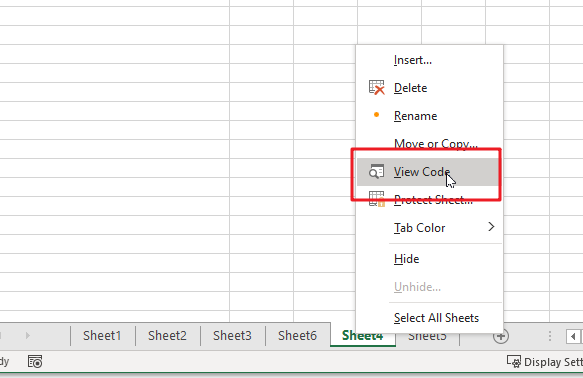Or you can enter Microsoft Visual Basic for Applications window via Developer->Visual Basic. You can also press Alt + F11 keys simultaneously to open it.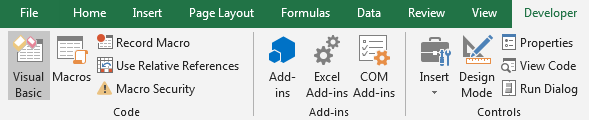Step 2: In Microsoft Visual Basic for Applications window, enter below code:

```Sub vlookupExample()

On Error Resume Next

lookupValue = "word"

Set myrange = Range("B2:C6")

result = Application.WorksheetFunction.VLookup(lookupValue, myrange, 2, False)

MsgBox "the sales number of " & lookupValue & " is " & result

End Sub```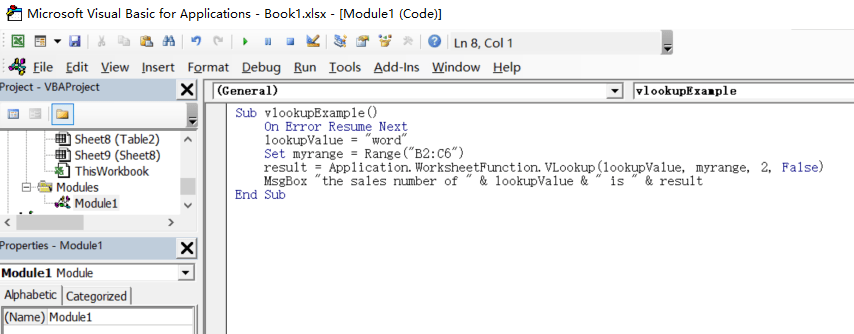Step 3: Save code, quit Microsoft Visual Basic for Applications.

Step 4: Click Developer->Macros to run Macro.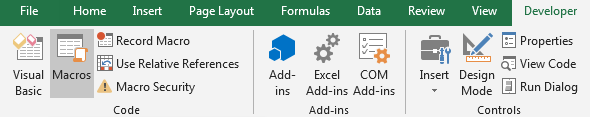Step 5: Select the Macro Name ‘vlookupExample’ from the Macro window and click Run.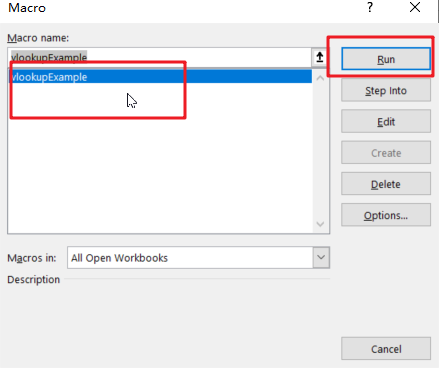Step6: let’s see the result: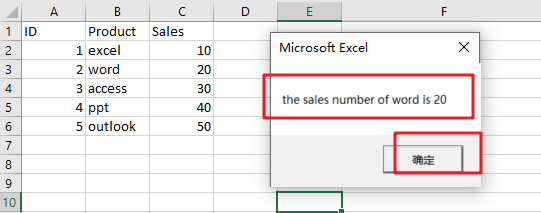Let’s see how this VBA Macro works:

You need to define a lookup value variable, which is the value to lookup.

lookupValue = “word”

Then you need to define the range in which the value and the return value exist. As your array table is B2:C6 in your worksheet, and defining a variable named as “myrange” as below:

Set myrange =Range(“B2:C6”)

Next, you need to call the VLOOKUP function from Application class and its sub-method WorksheetFunction, like below:

Application.WorksheetFunction.VLookup(lookupValue, myrange, 2, False)

Last, you can call MsgBox function to print the result in a message box, so you can use the following line:

MsgBox “the sales number of ” & lookupValue & ” is ” & result

### Related Functions

• Excel VLOOKUP function
The Excel VLOOKUP function lookup a value in the first column of the table and return the value in the same row based on index_num position.The syntax of the VLOOKUP function is as below:= VLOOKUP (lookup_value, table_array, column_index_num,[range_lookup])….

Sidebar